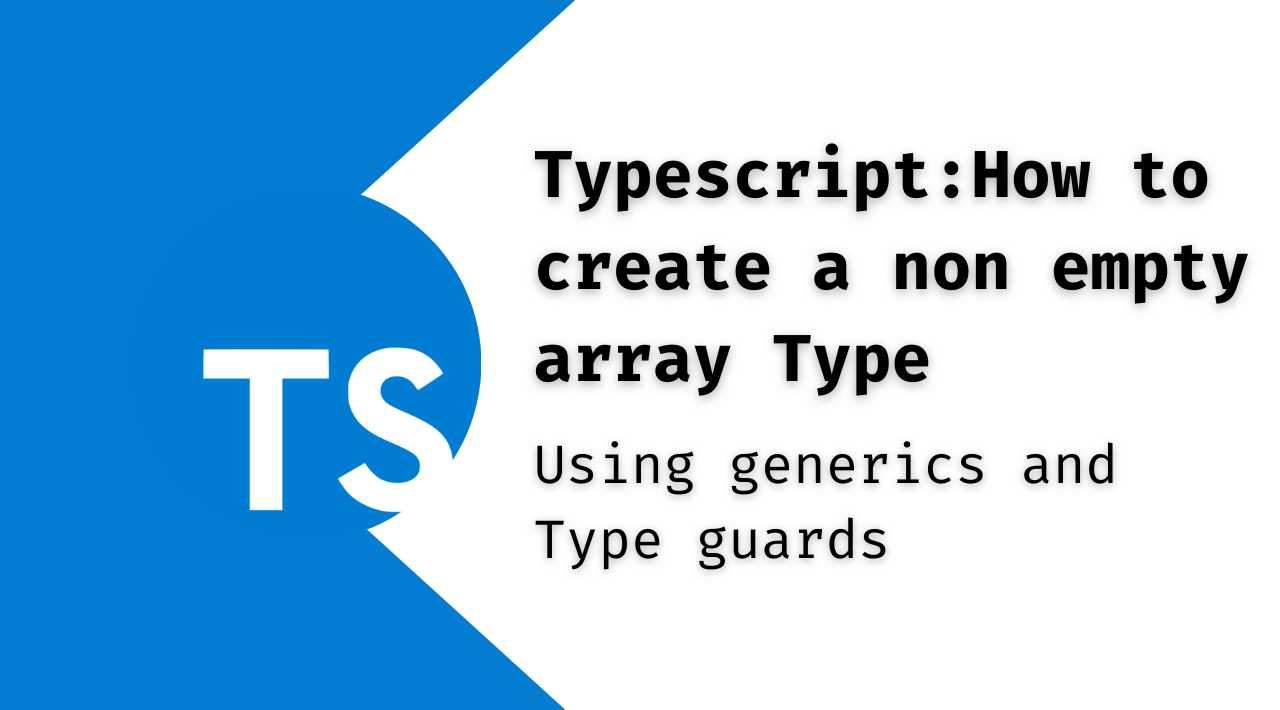## Typescript: How to create a non empty array Type

Typescript is a powerful and expressive language, which allows you to avoid publishing bugs.

Remember, Typescript is not just a "super set" over Javascript, it is a "Turing complete" language in its type system, that is, you can implement complex algorithms using only types.

But its power lies in how well you express the constraints of your application, that is, how well you define your types.

Typescript forces you to think differently when implementing a solution. You must think about how data flows through your program and how this information is transformed from "one form to another" That is, you must think about what types you will use.

One data type we use constantly is `Array`.

An array allows you to work with collections of data simply and efficiently.

In both Javascript and Typescript an array can be initialized just by using `[]` and you can define its type in a similar way or by using the generic form `Array<T>`

``````// An array that can contain any tipe and will be declared empty
const arr: unknown[] = []

// Another way to define the type
const arr2: Array<unknown> = []

// An array of strings defined with one element
const arr3: string[] = ['str']
``````

## How to avoid using empty arrays with Typescript?

An array can then be empty or contain `n` elements.

It is a common task to check whether or not an array is empty to operate on it. How can you determine if an array is empty?

In Javascript, this task is done with a conditional block and checking the `.length` property of the array.

But is it possible to use Typescript's typing language to prevent an array from being empty without using a conditional?

The idea here is to let Typescript check the data stream and give us an error if you're trying to access an empty array.

What you will do is create a new type similar to `Array` that allows you to define an array that cannot be empty by definition.

Let's call this type `NonEmptyArray`.

``````type NonEmptyArray<T> = [T, ...T[]]

const emptyArr: NonEmptyArray<Item> = [] // error ❌

const emptyArr2: Array<Item> = [] // ok ✅

function expectNonEmptyArray(arr: NonEmptyArray<unknown>) {
console.log('non empty array', arr)
}

expectNonEmptyArray([]) // you cannot pass an empty array. ❌

expectNonEmptyArray(['som valuue']) // ok ✅
``````

So whenever you require, for example, a function parameter to be an array that cannot be empty, you can use `NonEmptyArray` .

The only drawback is that you will now require a "type guard" function since simply checking if the `length` property of an array is not 0 will not transform it to type `NonEmptyArray`

``````function getArr(arr: NonEmptyArray<string>) {
return arr;
}

const arr3 = ['1']
if (arr3.length > 0)) {
// ⛔️ Error: Argument of type 'string[]' is not
// assignable to parameter of type 'NonEmptyArr<string>'.
getArr(arr3);
}
``````

This error occurs because `getArr` expects the argument to be `NonEmptyArray`, but `arr3` is of type `Array`.

#### Type guards

A "type-guard" function allows you to "help" Typescript correctly infer the type of some variable.

It is a simple function that returns a boolean value. If this value is `true` then Typescript will consider the variable evaluated to be one type or another.

``````// Type Guard
function isNonEmpty<A>(arr: Array<A>): arr is NonEmptyArray<A>{
return arr.length > 0
}
``````

This function receives a generic array (hence the use of `A`), and checks to see if the `length` property is greater than `0`.

This function is marked to return `arr is NonEmptyArray<A>` i.e. that the value of the condition evaluated is `true` Typescript will understand that the parameter to use `arr` is of type `NonEmptyArray`

``````// Type Guard
function isNonEmpty<A>(arr: Array<A>): arr is NonEmptyArray<A>{
return arr.length > 0
}

function getArr(arr: NonEmptyArray<string>) {
return arr;
}

const arr3 = ['1']
//     ^?   const arr3: string[]
if (isNonEmpty(arr3)) {
getArr(arr3);
//        ^? const arr3: NonEmptyArray<string>
}
``````

A simple way to understand type guard is that you "cast" one type to another type if and only if a certain condition is met. What makes this transformation safe compared to a simple type cast `as NonEmptyArray`

Check out the typescript playground with these examples.